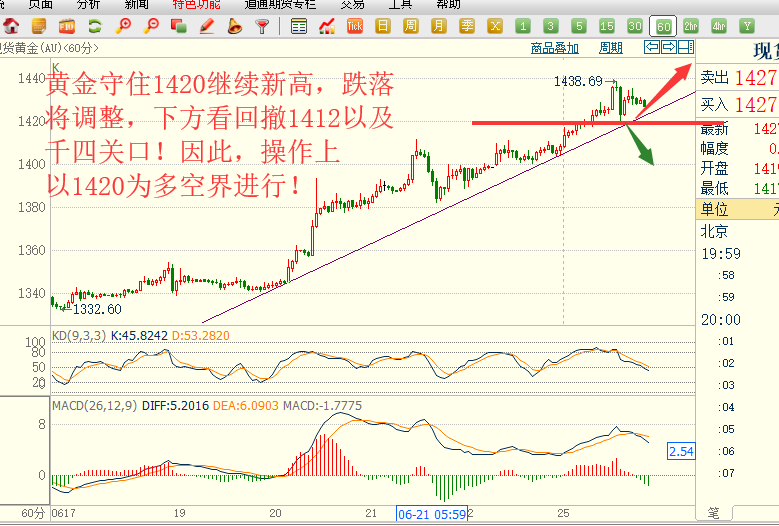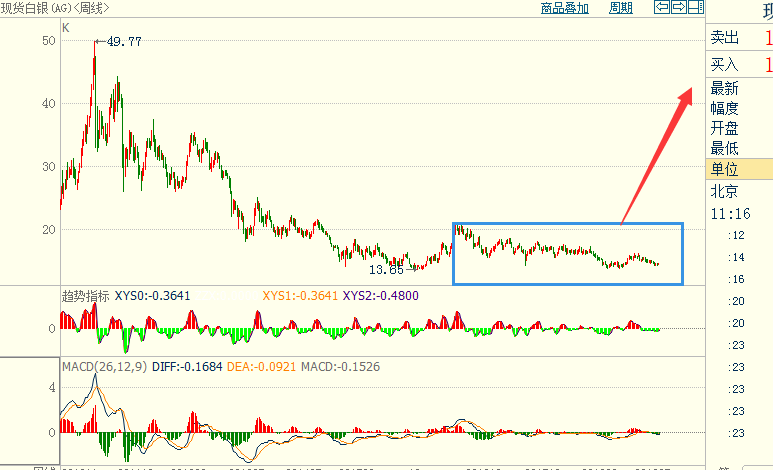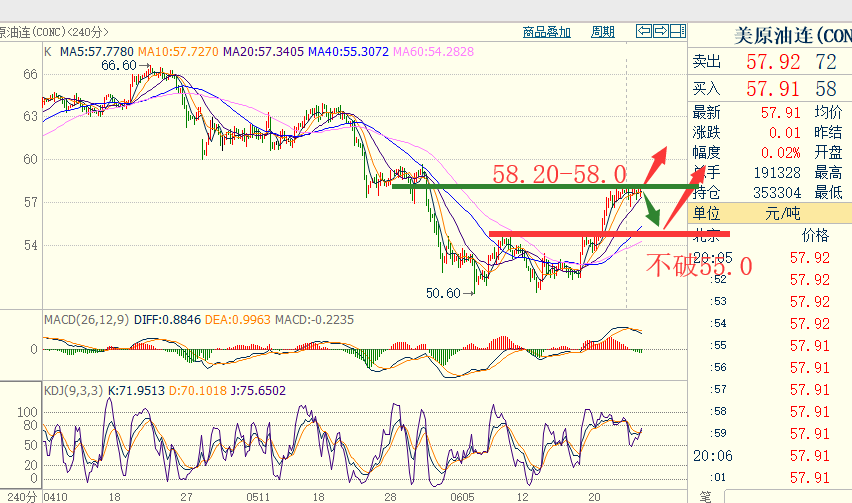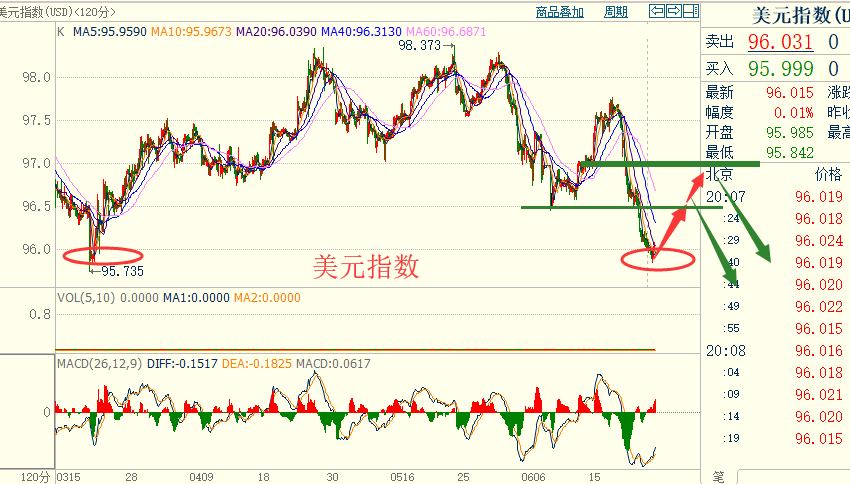杨朋威：黄金盯紧1420多空界，白银值得拥有！

时间:2019-06-25 21:47　来源：未知手机版500)this.width=500' align='center' hspace=10 vspace=10 rel='nofollow'/>500)this.width=500' align='center' hspace=10 vspace=10 rel='nofollow'/>500)this.width=500' align='center' hspace=10 vspace=10 rel='nofollow'/>500)this.width=500' align='center' hspace=10 vspace=10 rel='nofollow'/>